#Function Repository Resource:

# TriangularSpiralPoints

Get the coordinates of the points on a triangular spiral

Contributed by: George Beck
 ResourceFunction["TriangularSpiralPoints"][n] gives the list of coordinate pairs on an equilateral triangular spiral with n sides starting at the origin.

## Details and Options

The list starts at the origin and goes (roughly) NW, SW, E and repeats.

## Examples

### Basic Examples

Here are the points on the first four legs of the spiral:

 In:=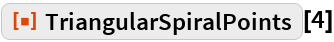Out=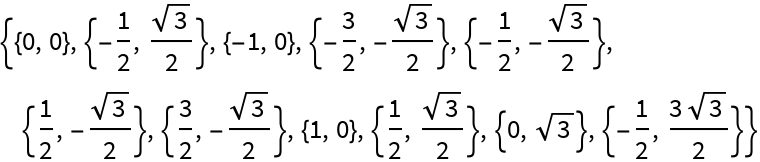This shows the sequence of points in order for the first six sides:

 In:=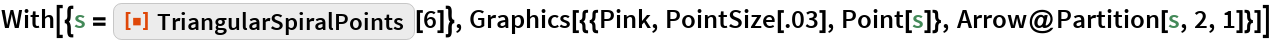Out=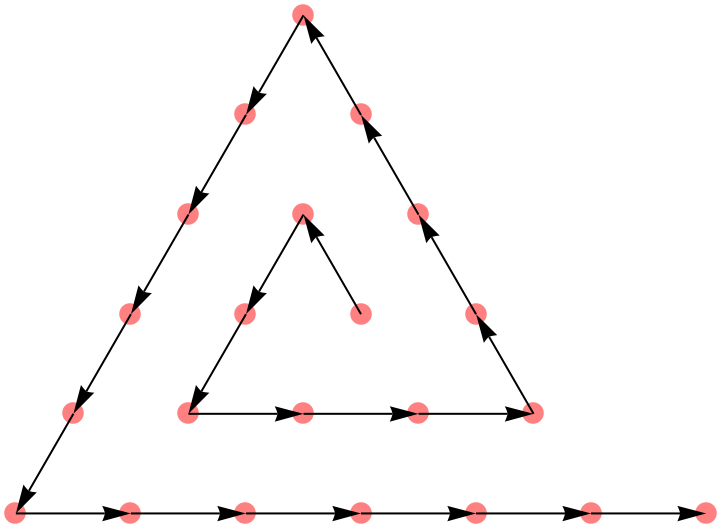40 black sides with 20 red sides overlaid:

 In:=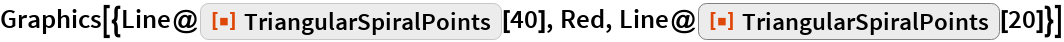Out=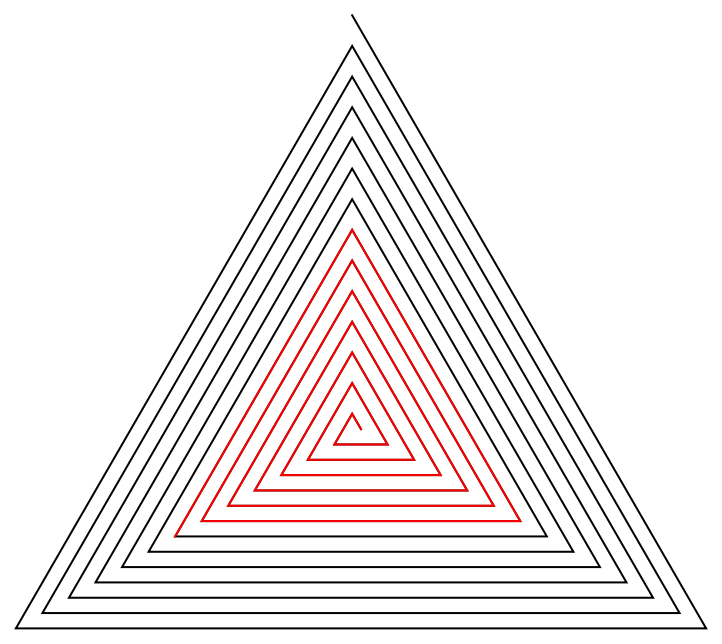The number of points in the first n sides are 1 more than the triangular numbers:

 In:=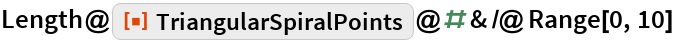Out=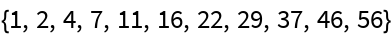In:=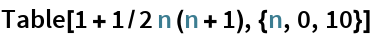Out=### Neat Examples

This finds the coordinate pairs that are a prime distance along the square spiral:

 In:=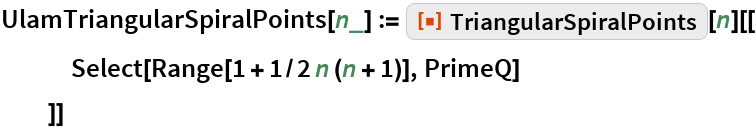The larger points correspond to the primes 2, 3, 5, 7, 11:

 In:=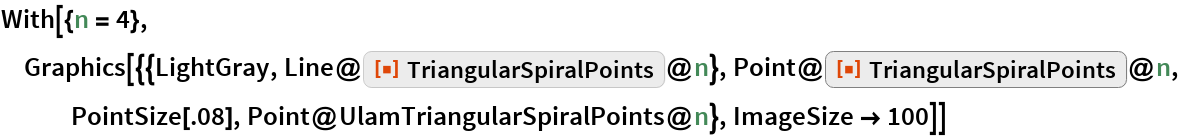Out=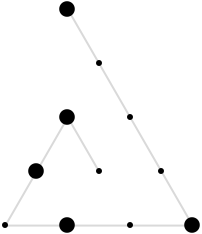About 12% of the numbers up to 11326 are prime:

 In:=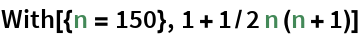Out=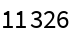In:=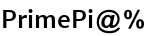Out=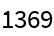Here is a plot of the first 1369 primes:

 In:=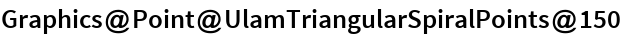Out=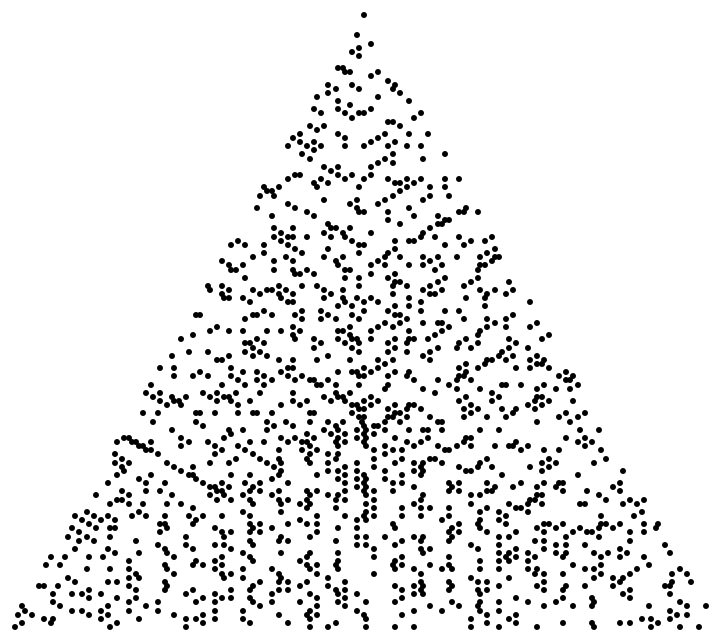About 11% of the numbers up to 11326 are lucky:

 In:=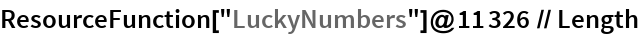Out=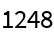This finds the coordinate pairs that are at lucky number distances along the triangular spiral:

 In:=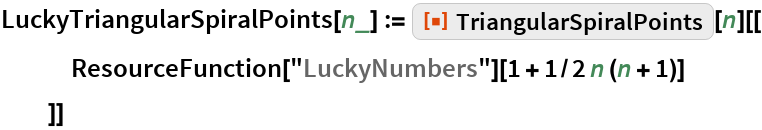Here are the first 1248 lucky numbers plotted along the triangular spiral:

 In:=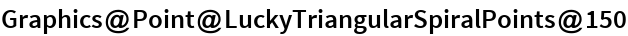Out=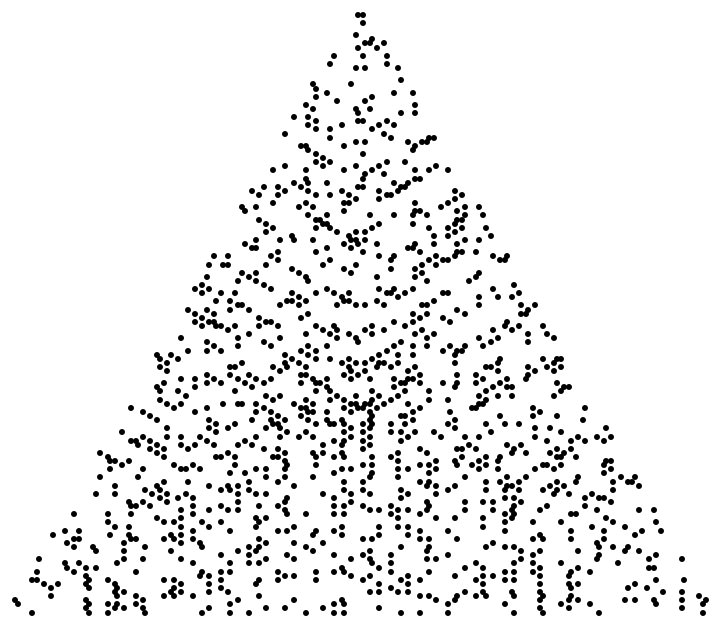## Requirements

Wolfram Language 11.3 (March 2018) or above